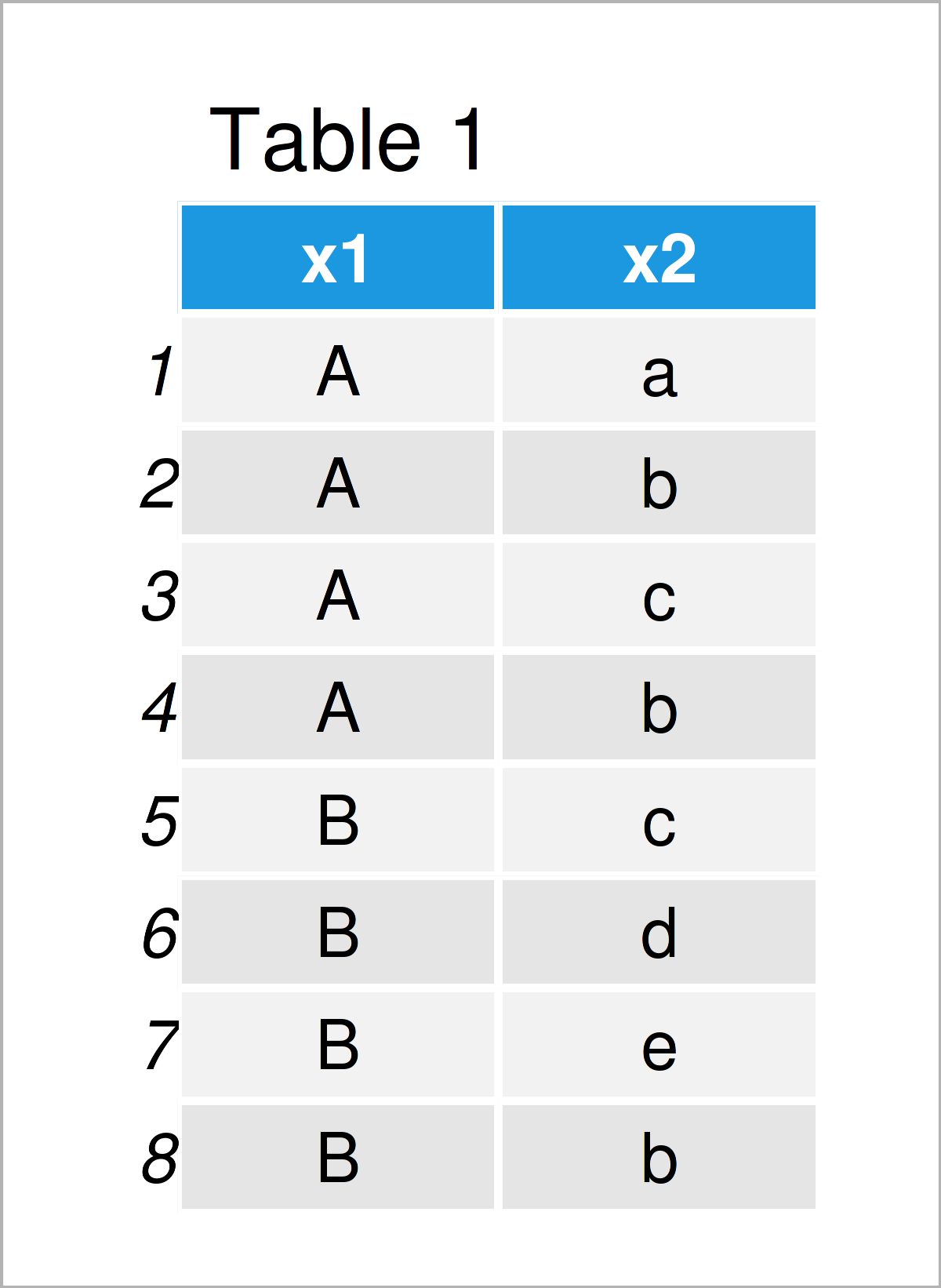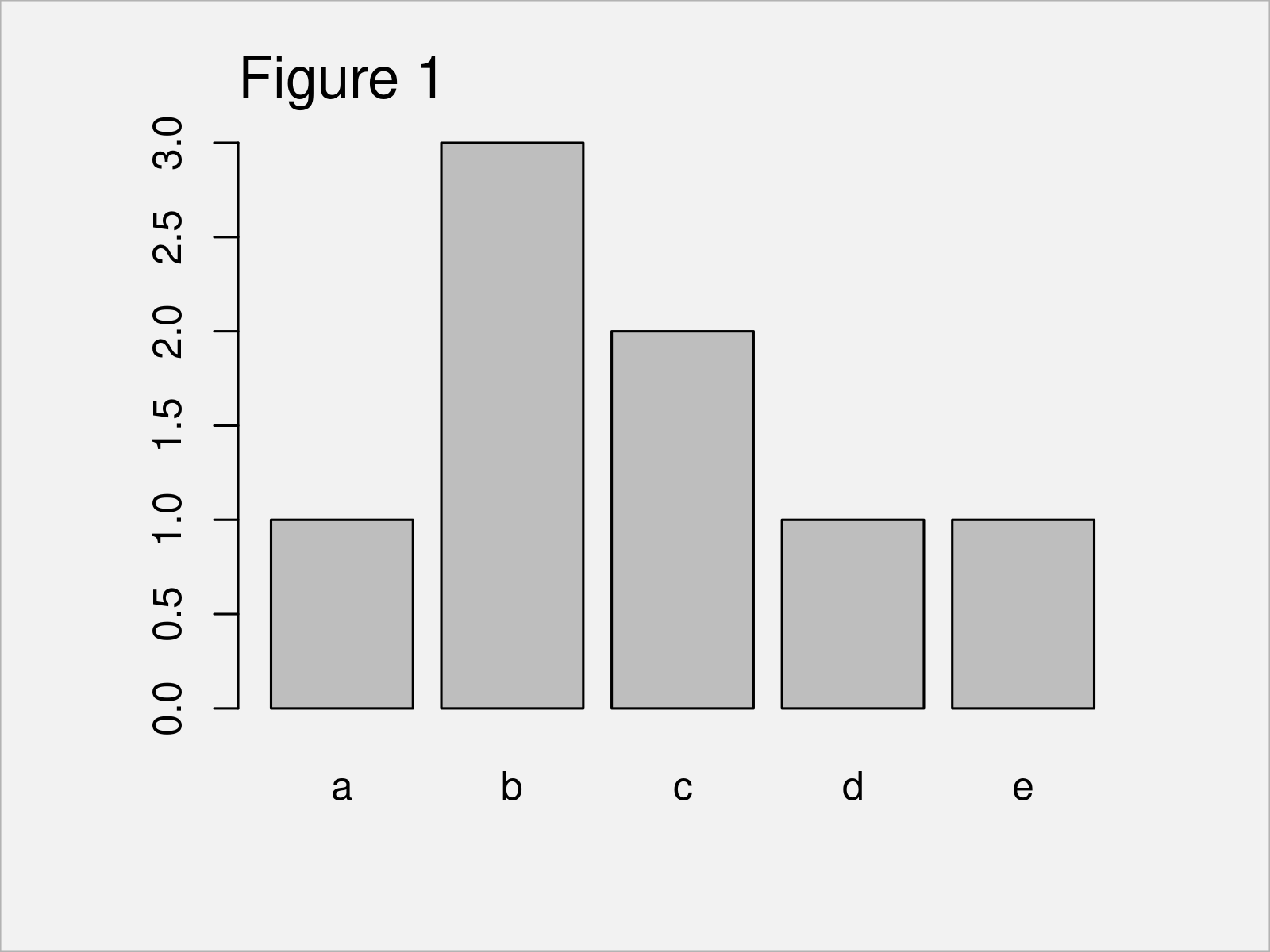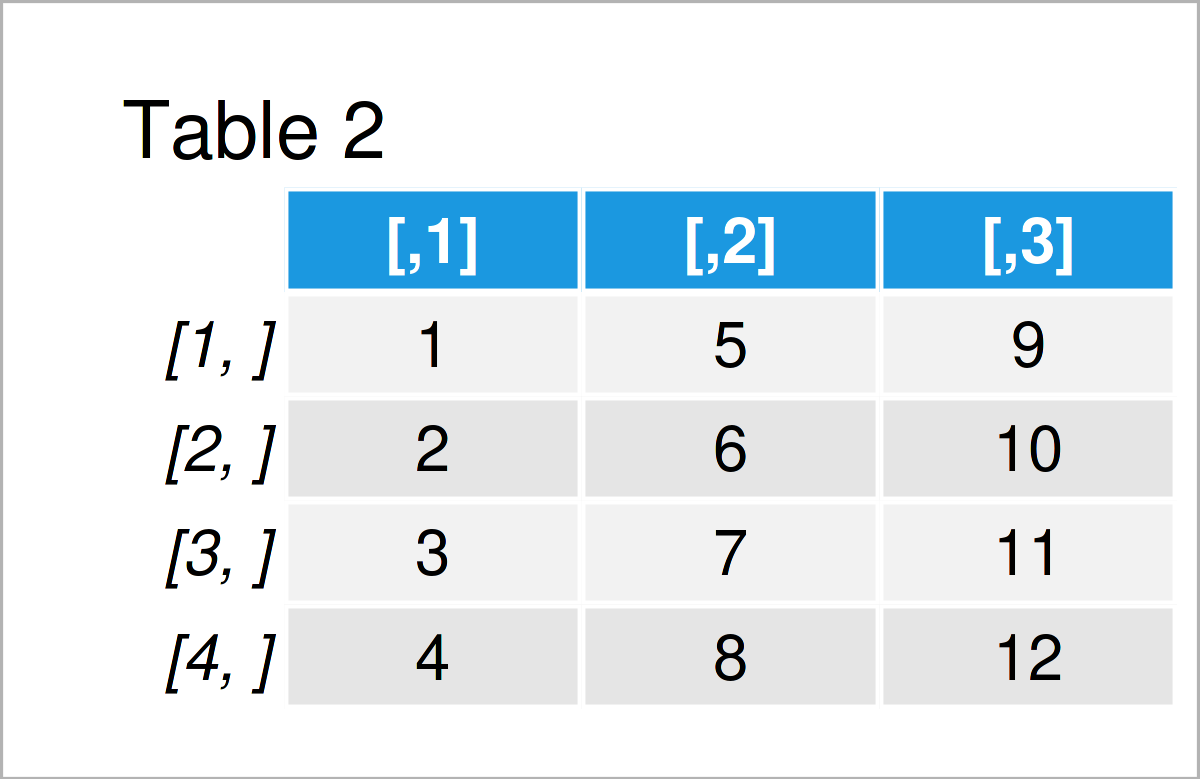# How to Create Tables in R (9 Examples)

In this R programming tutorial you’ll learn how to create, manipulate, and plot table objects.

The content of the page is structured as follows:

Note that this tutorial gives a brief overview on the usage of the table function in R. However, I have also published more detailed tutorials on the different topics shown in this tutorial. You may access these tutorials by clicking on the links within the corresponding sections.

Anyway, it’s time to dive into the programming part.

## Example Data

The following data will be used as basement for this R programming language tutorial:

```data <- data.frame(x1 = rep(LETTERS[1:2],       # Create example data frame
each = 4),
x2 = c(letters[1:3],
letters[2:5],
"b"))
data                                            # Print example data frame```Table 1 visualizes the output of the RStudio console and shows the structure of our exemplifying data – It is constituted of eight rows and two character columns.

## Example 1: Create Frequency Table

This example shows how to make a frequency table in R.

For this task, we can apply the table() function to one of the columns of our example data frame:

```tab1 <- table(data\$x2)                          # Make frequency table
tab1                                            # Print frequency table
# a b c d e
# 1 3 2 1 1```

The previous output shows the frequency counts of each value in the column x2. For instance, the letter a is contained once, and the letter b is contained three times.

## Example 2: Create Contingency Table

The following R programming code explains how to make a contingency table, i.e. a table of multiple columns.

The following R code creates a two-way cross tabulation of our example data frame:

```tab2 <- table(data)                             # Make contingency table
tab2                                            # Print contingency table
#    x2
# x1  a b c d e
#   A 1 2 1 0 0
#   B 0 1 1 1 1```

The previous output shows the frequency distribution among the two columns x1 and x2. For instance, the combination of A and a occurs once, and the combination of B and a appears not at all.

## Example 3: Sort Frequency Table

This example explains how to order a table object.

For this example, we use the table object tab1 that we have created in Example 1 as basis.

We sort this table by applying the order function. Within the order function, we set the decreasing argument to be equal to TRUE, to show the values with the most occurrences first.

Have a look at the following R code:

```tab3 <- tab1[order(tab1, decreasing = TRUE)]    # Order table
tab3                                            # Print ordered table
# b c a d e
# 3 2 1 1 1```

As you can see, the character b is shown first, since it occurs the most often in the data frame variable x2.

## Example 4: Change Names of Table

In Example 4, I’ll demonstrate how to rename the elements of a table.

For this, we can apply the names and paste0 functions as illustrated in the following R code:

```tab4 <- tab3                                    # Duplicate table
names(tab4) <- paste0("x", 1:length(tab4))      # Change names
tab4                                            # Print renamed table
# x1 x2 x3 x4 x5
#  3  2  1  1  1```

The previous output contains the same numeric values as the table that we have created in Example 3. However, the labels of those table cells have been changed.

## Example 5: Extract Subset of Table

The code below shows how to return only a certain subset of a table object.

To achieve this, we use the table object tab1 that we have constructed in Example1 as basis. We can select a subset of this table object using a logical condition as shown below:

```tab5 <- tab1[tab1 > 1]                          # Extract table subset
tab5                                            # Print table subset
# b c
# 3 2```

The previously shown table subset consists of all table elements that occur at least two times. All the other table elements have been removed.

## Example 6: Create Proportions Table

In Example 6, I’ll explain how to create a proportions table (or probabilities).

For this task, we can apply the prop.table command to a table object (i.e. tab1) as illustrated in the following R syntax:

```tab6 <- prop.table(tab1)                        # Make proportions table
tab6                                            # Print proportions table
#     a     b     c     d     e
# 0.125 0.375 0.250 0.125 0.125```

The previous output shows the proportions of each value in our data.

## Example 7: Draw Table in Barplot

In Example 7, I’ll show how to plot a table object in a barchart.

To do this, we have to apply the barplot function to a table object:

`barplot(tab1)                                   # Draw table in plot`Figure 1 shows the output of the previous R code: A Base R bargraph showing the values in the table we have created in Example 1. The height of the bars corresponds to the occurrences of each value in our data set variable.

## Example 8: Convert Matrix to Table

This example explains how to change the data type of a numeric matrix object to the table class.

For this example, we first have to create an exemplifying matrix:

```mat <- matrix(1:12, ncol = 3)                   # Create example matrix
mat                                             # Print example matrix```As shown in Table 2, the previous R programming code has created a matrix object with four rows and three columns.

We can now use the as.table function to convert this matrix to the table class:

```tab7 <- as.table(mat)                           # Convert matrix to table
tab7                                            # Print converted table
#    A  B  C
# A  1  5  9
# B  2  6 10
# C  3  7 11
# D  4  8 12```

The previous output shows our new table object that we have created based on our input matrix.

## Example 9: Check Class of Table Object

This example illustrates how to check whether a data object has the table class.

There are basically two alternatives on how to do this. Either, we can apply the class() function to return the class of a data object

```class(tab7)                                     # Return class of table
#  "table"```

…or we can apply the is.table function to return a logical indicator that shows whether our data object has the table class:

```is.table(tab7)                                  # Test if object is table
#  TRUE```

Both applications return the same result: The data object tab7 that we have created in Example 8 has the table class.

## Video, Further Resources & Summary

In case you need further explanations on the examples of this tutorial, you might want to have a look at the following video on my YouTube channel. I’m showing the content of this article in the video.

In addition, you may want to have a look at the other articles on my website. I have created a tutorial series that contains many additional instructions on how to use tables in R:

Summary: At this point of the article you should have learned how to apply the table command to calculate, construct, work, modify, and draw table objects in R programming. In case you have additional questions, don’t hesitate to tell me about it in the comments below.

Subscribe to the Statistics Globe Newsletter

•ALI
March 9, 2022 8:45 am

Dear Joachim, Thanks for the great work!
Could you please guide me on how to find the performance of the Bayesian Moving Average control chart using Posterior/prior distribution through ARL and SDRL as performance measures with the help of Monte Carlo Simulations?

•March 10, 2022 8:24 am

Hey Ali,

Thank you for the kind comment! Unfortunately, I’m not an expert on this topic. However, I have recently created a Facebook discussion group where people can ask questions about R programming and statistics. Could you post your question there? This way, others can contribute/read as well: https://www.facebook.com/groups/statisticsglobe

Regards,
Joachim

•Hussein
March 26, 2023 1:40 pm

thanks that was very useful

•March 27, 2023 10:26 am